Function Repository Resource:

# InflectionPoints

Find the inflection points of a function of one variable

Contributed by: Wolfram|Alpha Math Team
 ResourceFunction["InflectionPoints"][expr, x] computes the inflection points of the expression expr with respect to variable x. ResourceFunction["InflectionPoints"][{expr,constraint},x] computes the inflection points expr, subject to the given condition constraint on x. ResourceFunction["InflectionPoints"][…, "Properties"] computes the inflection points of a function, along with function properties at these points.

## Details and Options

Inflection points are returned as a list of rules for the independent variable x. When the "Properties" directive is invoked, inflection points are listed along with properties such as "rising", "falling", "stationary" and "non-stationary".
For functions with a repeating pattern of inflection points, ResourceFunction["InflectionPoints"] returns results in terms of one or more undetermined constants,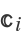which can take any integer value.
In ResourceFunction["InflectionPoints"][{expr,constraint},x], the constraint argument should be an inequality involving x.

## Examples

### Basic Examples (4)

Find the inflection points of a cubic function:

 In:=Out=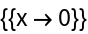Plot the function and its inflection points found above:

 In:=Out=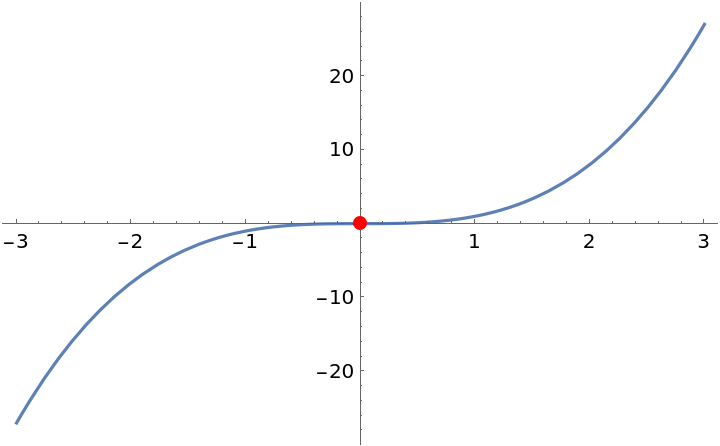Repeat the calculation, classifying inflection points:

 In:=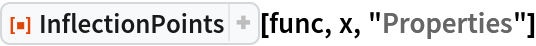Out=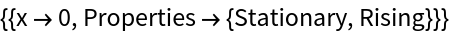Find and classify the inflection points of a polynomial function:

 In:=Out=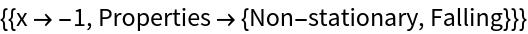Plot the function and its inflection points:

 In:=Out=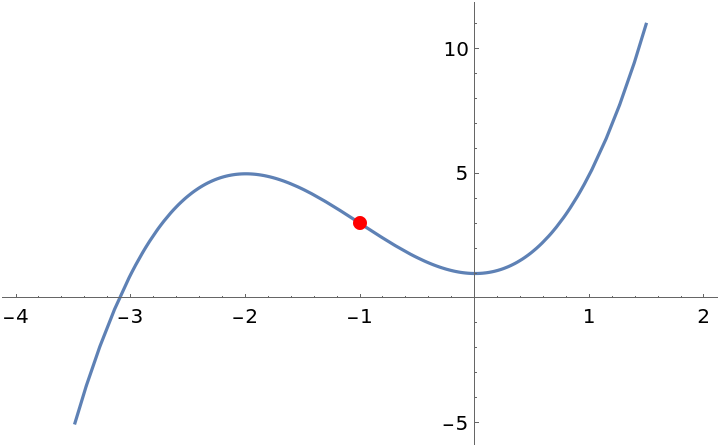Find and classify the inflection points of another polynomial function:

 In:=Out=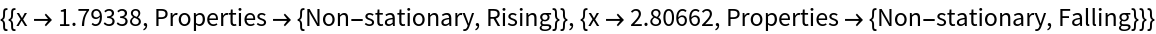Plot the function and its inflection points:

 In:=Out=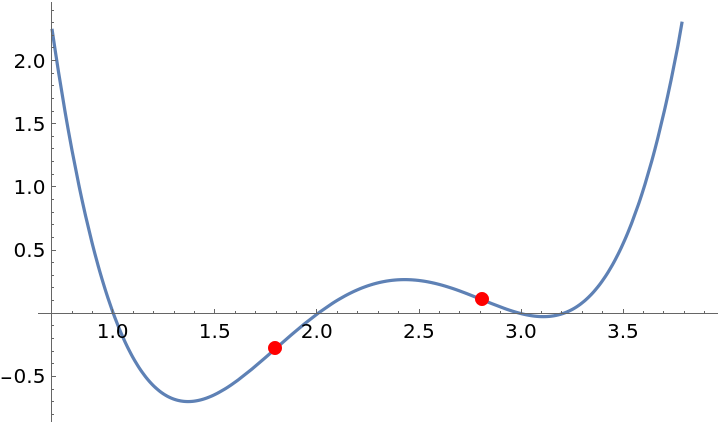Find and classify the inflection points of a trigonometric function:

 In:=Out=Plot the function and a single cycle's worth of its inflection points:

 In:=Out=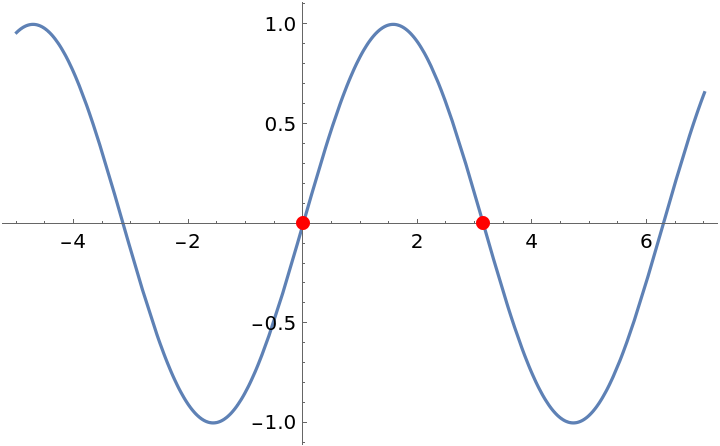### Options (2)

Find and classify the inflection points of a function, specifying the range of the independent variable to include:

 In:=Out=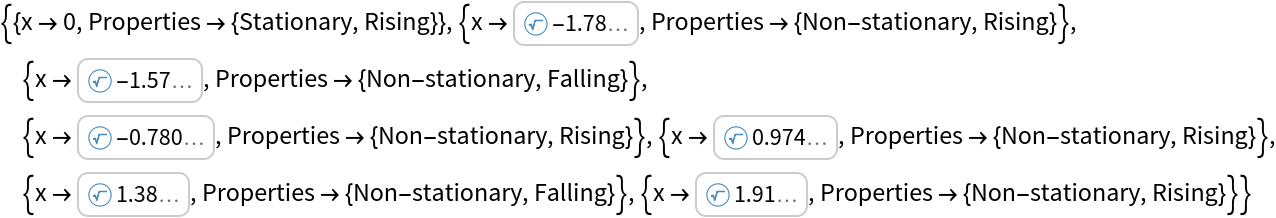Plot the function and its inflection points:

 In:=Out=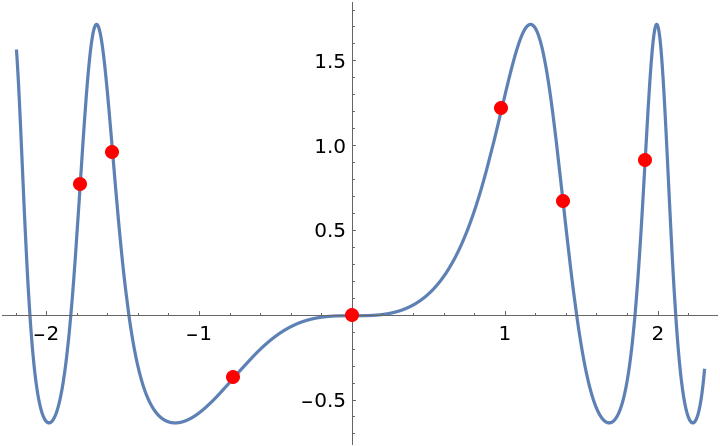### Properties and Relations (3)

InflectionPoints will sometimes return results in terms of Root objects:

 In:=Out=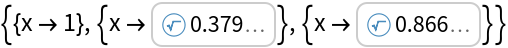Applying N to these outputs converts to an ordinary numeric result:

 In:=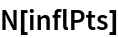Out=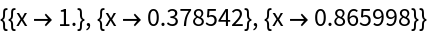Plot the function and its inflection points:

 In:=Out=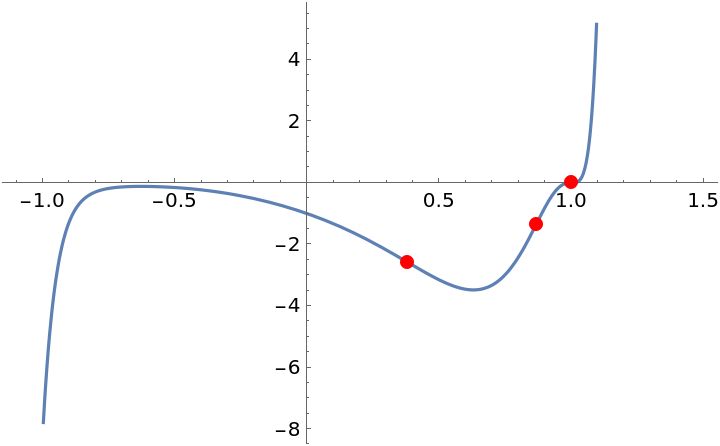For functions with a repeating pattern of inflection points, InflectionPoints returns results in terms of one or more undetermined constants, which can take any integer value:

 In:=Out=Plot the function and a single period's worth of its inflection points:

 In:=Out=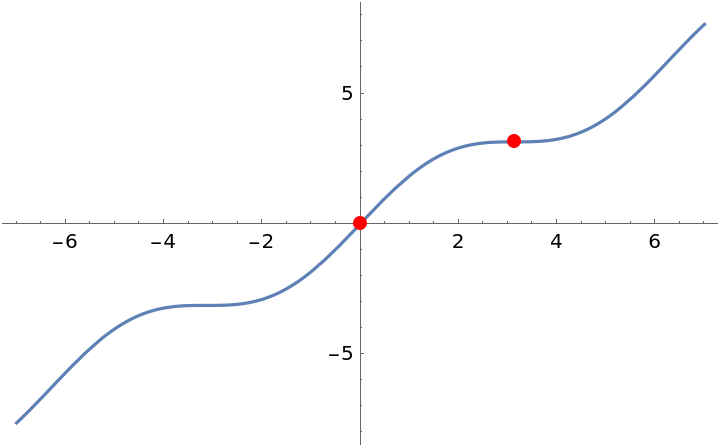Find the inflection points of another periodic function:

 In:=Out=Plot the function and a single cycle's worth of its inflection points:

 In:=Out=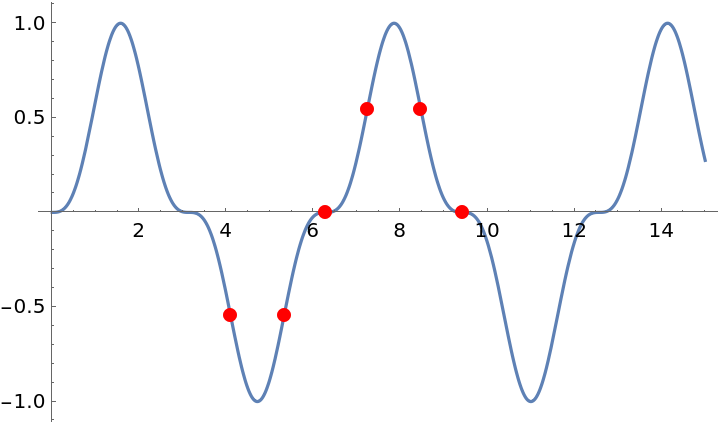## Publisher

Wolfram|Alpha Math Team

## Version History

• 5.0.0 – 23 March 2023
• 4.0.0 – 01 April 2020
• 3.0.0 – 06 September 2019
• 2.0.0 – 12 June 2019
• 1.0.0 – 22 February 2019